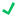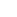# How to Calculate Inclusive Tax

Modified on: Thu, 19 Sep, 2019 at 8:37 AM

Applies to:Rover PMSRover Overlay

# How to Calculate Inclusive Tax

Inclusive tax is calculated assuming the net amount is 100%, not the gross amount. In a more simplistic example, if the rate is \$100, and tax is 20%, you will have to divide 100 by (120 x 20) to get to the correct tax amount, meaning tax is 16.67% and not 20%. See the example below for functionality.

Room rate: \$124

Base amount: \$104.98 (calculated by considering the following 4 inclusive taxes added in the charge code 160 - Room Charge - Tax Inclusive)

4 inclusive taxes:

• Tax 1 Charge Code: 820 (amount \$2), inclusive tax = \$2
• Tax 2 Charge Code: 810 (5.875%), inclusive tax = 5.875% of \$104.98 = \$6.17
• Tax 3 Charge Code: 800 (8.875%), inclusive tax = 8.875% of \$104.98 = \$9.32
• Tax 4 Charge Code: 830 (\$1.5), inclusive tax = \$1.5

Based on the above, a total of \$18.99 will be displayed as FEES in the deposit balance.

Did you find it helpful? Yes No

Send feedback